# Math 1050 - MAPLE LAB 1

Maple Lab I

## Math 1050 - Precalculus - Introduction to Maple

Do this to start a session in Maple:

1. To turn on the computer press the space bar to wake it up, then press Ctrl & Alt & Delete simultaneously. Enter the username as "mathuser", and the password you were given in class, then click OK.

2. When Windows opens, use the mouse to point to the MAPLE V icon, then double click the right mouse button.

3. When Maple opens you will notice a prompt "[>" appearing in the top left hand corner of the white screen. This prompt indicates an input line. You can enter MAPLE commands at this prompt. Remember to end all command lines with a semicolon to execute the command. Then by pressing enter, the command will be performed and the output displayed in the center of the screen below the command line.

4. If you have any questions please do not hesitate to ask either the lab monitor on duty or an employee of the Math Learning Center located in ENGR 140.

A few examples of MAPLE command lines will follow for you to try, then a few exercises which you are to complete, print, and turn in to your instructor.

Examples:
To graph an equation, first solve the equation for y, then use the plot command in MAPLE to graph it. To graph the equation y=3x-4 displaying x values from x=-10 to x=10, the command should appear as below.  Note that you must always tell the computer what x values to use.  The command is followed by the MAPLE output of a graph.

> plot(3*x-4,x=-10..10);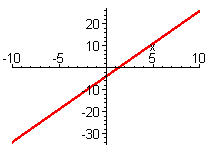*** Notice all multiplication must be entered using the * key, otherwise MAPLE will not recognize it as multiplication.

*** Also be sure to end each line with a semi-colon or MAPLE won't realize you've finished your request.

If you need to graph two or more equations on the same axis, then you need to use braces to let MAPLE know there are multiple equations present. To graph the equations y= x3+5x and y=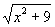, displaying from -3 to 3 on the x-axis, the command would list the set of equations in braces separated by commas as follows:

*** Notice to enter exponents the ^ symbol is used.

> plot({x^3+5*x,sqrt(x^2+9)},x=-3..3);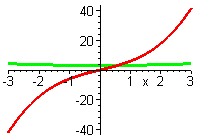A square root can also be done using an exponent of 1/2 as shown below:
> plot({x^3+5*x,(x^2+9)^(1/2)},x=-3..3);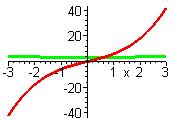Observe that both of the commands above produce the same graph.

However, the y values on this graph are so large it is difficult to see details, in order to zoom in on the graph we can declare the y values that will be displayed as well. So let's see if narrowing down the y values to between y=-5 and y=5, (or -5 < y < 5) helps to clarify the picture. The command for this just adds the y-values we want to see after the x-values.

> plot({x^3+5*x,sqrt(x^2+9)},x=-3..3,y=-5..5);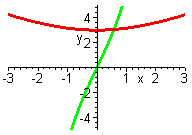There is another method that may be used to graph functions. This method involves naming (or defining) the functions. You can name these functions using the arrow notation in MAPLE "->" which is made by a dash followed by the greater than sign. This also allows MAPLE to evaluate and manipulate these functions. For example, to name the equation y=2x3-1 as f(x)=2x3-1  and to name g(x)=7x-2. The command to name these in MAPLE is entered as follows.

> f:=x->2*x^3-1;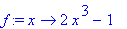> g:=x->7*x-2;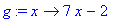MAPLE reads this command as though you defined a function g to map the variable x onto 7x-2. However, you must be extremely careful when naming functions and double check your output because, if any character is left out MAPLE will not recognize your function. Also note that if the name f is ever used again MAPLE will use the new function as f and forget this one.

Now in order to graph both of these functions on the same graph without rewriting the equations, the command becomes:

> plot({f(x),g(x)},x=-5..7);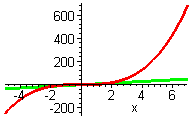Now we want to define new functions,  h(x)=x4 and g(x)=2x3-3.  Note that the old function under the name g will be lost, other names may be used as well if you do not want to lose the g already defined, even strings of letters and numbers may be used as names..

> h:=x->x^4;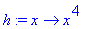> g:=x->2*x^3-3;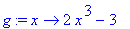Now that the functions are defined, operations may be performed on them. To find the value of h(x) at x=-13, and g(x) at x=2 the commands are below. However MAPLE is not limited to numerical evaluations. The algebraic expression of g(t-3) can also be obtained easily.

> h(-13);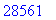> g(2);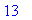> g(t-3);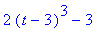The algebraic representation of g(t-3) has been found, but what if you need to collect terms or remove parenthesis? Well you can ask MAPLE to simplify the expression for you, as directed below.

> simplify(g(t-3));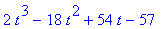The exercises below are to be completed, printed and turned into your instructor (both the commands you enter and the MAPLE output should be turned in). If you have a disk you may also want to save your work for questions or later completion if necessary. If you completed the examples and wish to start the exercises on a clear screen, go to the start of the document with the mouse, hold down the right button and drag it to the bottom so that it highlights what you would like to delete. Release the mouse button and press delete.

Saving : To save your work to your disk, go to the file menu and select save. Be sure to change the drive selection to the a: drive, then type in the name you would like to save it under and click on OK.

Printing : When you are ready to print, it is best to access print through the file menu as well. This way you have the option of selecting a printer in your room. If you are in the lab located in ENGR 136 select the printer named EAS136, in room ENRGR 138 select the printer named EAS138, then click on OK.

Exercises:

1. On the same graph plot the equations y=2x2-3x+10 and y=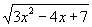on the interval
-3 < x < 5.

2. Repeat the graphs of problem #1 but, this time, display the graphs on the intervals 0 < x < 30 for the x axis and -10 < y < 100 for the y axis.

3. For f(x)=3x2-2x+1 and g(x)=4x+5 and complete a-g.
a. Use MAPLE to define the functions.
b. Graph them on the same axis using their names over the intervals -5 < x <5 and -10 < y < 10
c. Evaluate f(31)
d. Evaluate g(-112)
e. Find g(3-x)
f. Find and then simplify f(x-2)